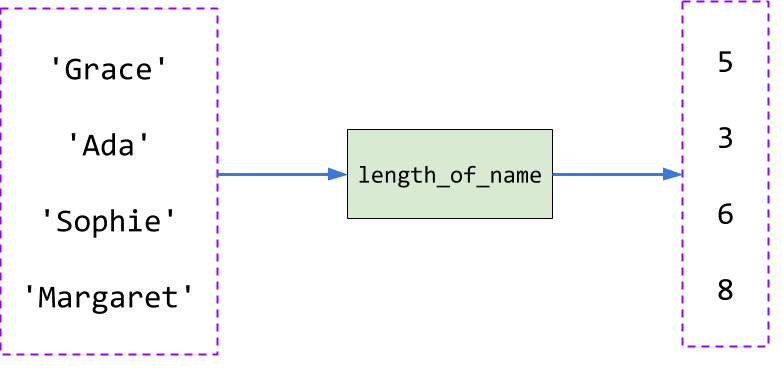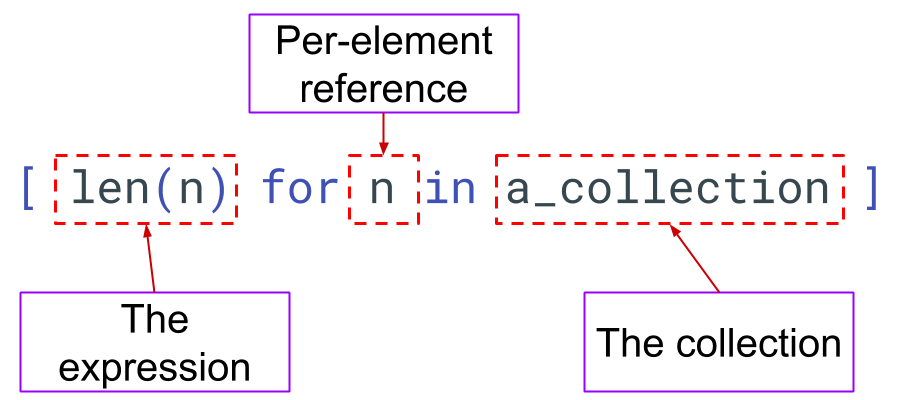# List Comprehensions Explained

Last updated: February 24th, 2020

Do you want to learn about List Comprehensions? What are they used for? How to write them?

We've recorded a detailed video with all these explanations, along with some tips to help you remember the syntax.

Important! You can fork this project and work on these examples and the exercises included by using the Fork button on the right 👉

(Desktop recommended)

In :
from IPython.display import YouTubeVideo

Out:

The objective of a List Comprehension is to turn a collection A into a collection of B. To do that, we define a transformation function which takes an element of A and transform it into an element of B. For example, we have a list of names:

In :
names = ['Grace', 'Ada', 'Sophie', 'Margaret']


and we want to "transform" it into a list of the lenght of each name. Our final result will look something like:

In :
[5, 3, 6, 8]

Out:
[5, 3, 6, 8]

### The Transform Function¶

To do that, we have to first define a function that receives a name, and returns its length in characters:

In :
def length_of_name(name):
return len(name)### From Collection A to Collection B¶

As you've seen, we've defined the function length_of_name to work only on one element of A. The list comprehension will be the one in charge of applying that function to every element of A and create a new collection.As you can see, the transformation is applied in order.

### List Comprehension Syntax¶

we'll transform this code using a list comprehension now, I'll just show it first and we can dissect it later:

In :
[length_of_name(name) for name in names]

Out:
[5, 3, 6, 8]

Another example:

In :
[length_of_name(n) for n in names]

Out:
[5, 3, 6, 8]

We can see that a list comprehension has 3 clearly defined parts:1. The expression: how would you "transform" each element in the collection
2. A name we're choosing to reference each element in the collection
3. The collection we want to transform

If you read the previous examples again, you'll see that I switch the name of the variable (2) between name and n. You can pick any name that you want. We try it to be representative of the elements you're iterating ("self documented").

### Any expression is valid¶

You'll probably notice that the function length_of_name is just a wrapper for the len function. So we can just use the expression len(name):

In :
[len(n) for n in names]

Out:
[5, 3, 6, 8]

This is something that usually confuse new programmers. It's related to the concept of "expressions" (maybe the subject for another video? 😅). Here's another example:

In :
import math

In :
constants = [math.pi, math.e, math.tau]
constants

Out:
[3.141592653589793, 2.718281828459045, 6.283185307179586]
In :
[f"{constant:.2f}" for constant in constants]

Out:
['3.14', '2.72', '6.28']

what we're doing is just formatting the numbers including only 2 decimals. The key is in the expression f"{constant:.2f}". Let's separate this in just one example:

In :
ϕ = (1 + 5 ** 0.5) / 2  # Phi constant, or golden ratio
ϕ

Out:
1.618033988749895
In :
f"{ϕ:.4f}"

Out:
'1.6180'

Sorry about the tangent, but I know I used a not-so-common variable name. In Python 3 we can use an extended range of unicode characters to name variables 😃. Maybe it's more clear with:

In :
golden_ratio = (1 + 5 ** 0.5) / 2  # Phi constant, or golden ratio
golden_ratio

Out:
1.618033988749895
In :
f"{golden_ratio:.4f}"

Out:
'1.6180'

But I digress... The important part is that we're using just an expression for our list comprehension. We could have used the regular format method:

In :
["{:.2f}".format(c) for c in constants]

Out:
['3.14', '2.72', '6.28']

### Immutability is key¶

One very important trait of List comprehensions is that they return a new collection, they DO NOT modify the original one. Using our previous example:

In :
[f"{constant:.2f}" for constant in constants]

Out:
['3.14', '2.72', '6.28']

But the list constants remains unchanged:

In :
constants

Out:
[3.141592653589793, 2.718281828459045, 6.283185307179586]

### Collection is actually any "iterable" object¶

List Comprehensions are not restricted to "just lists". They're called List comprehensions because they return lists, but any iterable can be the input of them. Check the following examples:

Using a dictionary:

In :
constants = {
"π": math.pi,
"e": math.e,
"τ": math.tau
}


Iterating over values:

In :
[f"{c:.2f}" for c in constants.values()]

Out:
['3.14', '2.72', '6.28']

Iterating over keys:

In :
[c.encode("unicode_escape") for c in constants]

Out:
[b'\\u03c0', b'e', b'\\u03c4']
In :
import numpy as np

In :
arr = np.arange(10)
arr

Out:
array([0, 1, 2, 3, 4, 5, 6, 7, 8, 9])
In :
[n ** 2 for n in arr]

Out:
[0, 1, 4, 9, 16, 25, 36, 49, 64, 81]

### The if part of list comprehensions¶

List comprehensions accept a final term which will let you "select" which elements will be processed. Syntactically, it's place at the end of the list comprehension. Check the following example, in which we run the expression n ** 2 ONLY for those elements that are divisible by 2:

In :
[n ** 2 for n in arr if n % 2 == 0]

Out:
[0, 4, 16, 36, 64]

As you can see, the if expression, is just any valid boolean expression.

In :
names = ['Grace', 'Ada', 'Sophie', 'Margaret']

In :
[name.upper() for name in names if len(name) > 3]

Out:
['GRACE', 'SOPHIE', 'MARGARET']

Sometimes, list comprehensions might incur in what's known as "double evaluation", as we can see in the following example, where we're trying to return the length of the names, but only for those names that have more than 3 characters:

In :
[len(name) for name in names if len(name) > 3]

Out:
[5, 6, 8]

In this case, len(name) was computed 2 times. If that operation is computationally expensive, we'll have a problem. There are ways of fixing this using generators, but that's the subject of other lesson 😁.

### The alternatives for Data Scientists¶

List Comprehensions are syntactic sugar for the glorious "map" operation in functional programming. Some other libraries have implemented the same logic with other mechanisms. The most important example is the one of "vectorized operations" in numpy and pandas. The same expression above could have been written in this way:

In :
arr ** 2

Out:
array([ 0,  1,  4,  9, 16, 25, 36, 49, 64, 81])

You can see that it yields the same results.

Vectorized operations are very important when working with numpy and pandas, and they're the preferred method (over List Comprehensions). They're also low-level optimized, so they'll result in better performance.

We've written extensively about vectorized operations and ufuncs. Check this post for an introduction.

### Next steps¶

We've seen a good overview of list comprehensions, how they're formed and what they are used for. If you want to keep investigating, there are some other topics that follow up to this, like Dictionary Comprehensions, Generators and Iterators.